# Newton's first law – examples

Example 1

Objects in motion stay in motion and objects at rest stay at rest unless acted upon by an outside force (unbalanced force).A stationary object with no outside force will not move.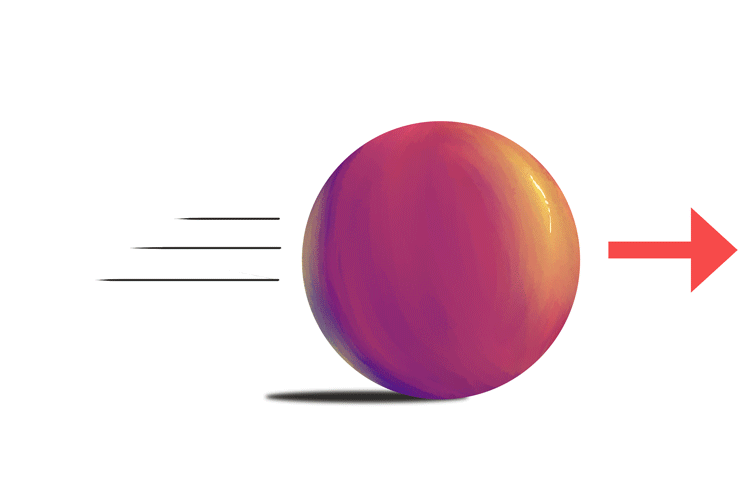With no outside forces, a moving object will not stop.

Although Sir Isaac Newton did not have access to space stations, this first law can clearly be seen:An astronaut who has their screwdriver knocked into space will see the screwdriver continue on at the same speed and direction forever. There are no forces acting on the screwdriver from friction. With no forces this object will never stop.

Example 2

Unless I am forced I do the same thing.An object at rest stays at rest.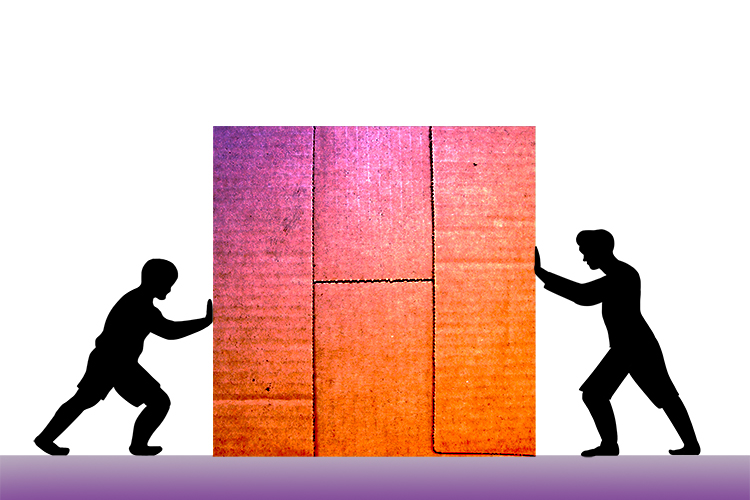An object acted upon by balanced forces stays at rest.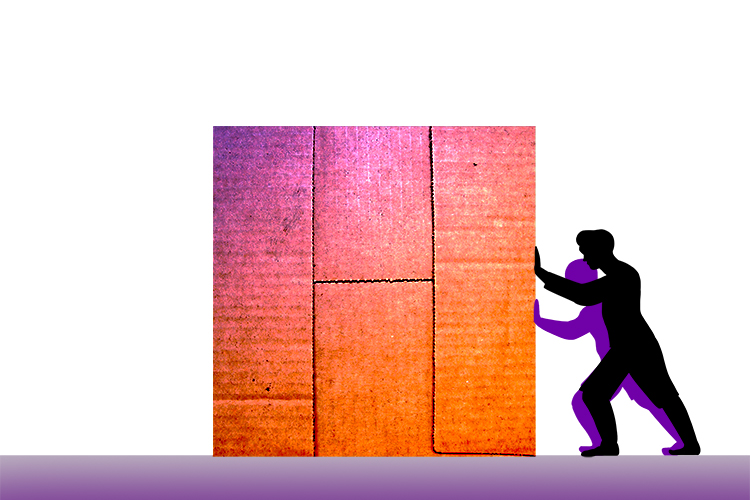An object acted upon by unbalanced forces changes speed and can change direction.

Example 3

Newton's first law is often referred to as the law of inertia – inertia is an object's tendency to resist changes in motion.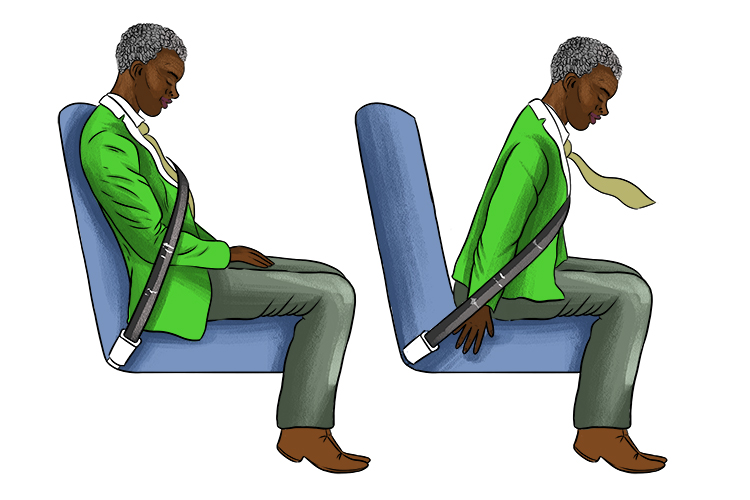When a car brakes quickly, the passenger will be thrown forward because inertia (the tendency to remain unchanged) tries to keep the passenger moving.

Example 4

The first law explains why you go flying over the handlebars if your bike stops suddenly: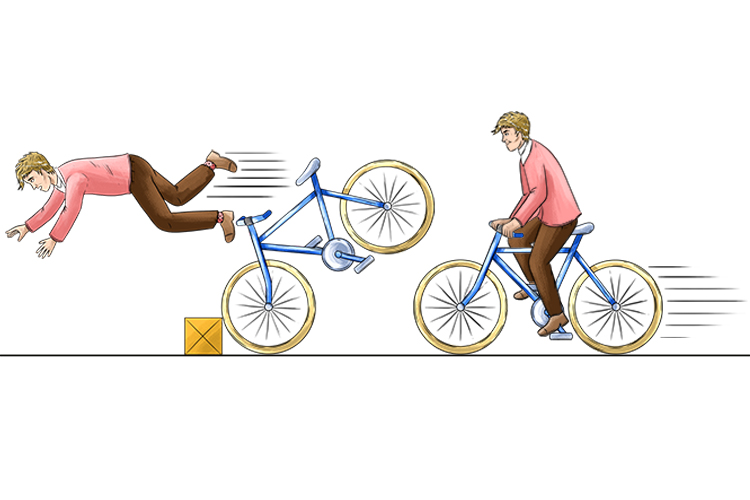Things tend to keep doing whatever they were doing before.

Example 5

If an object is stationary, it will remain stationary. If the object is moving it will continue to move at the same speed and in the same direction.An object at rest stays at rest.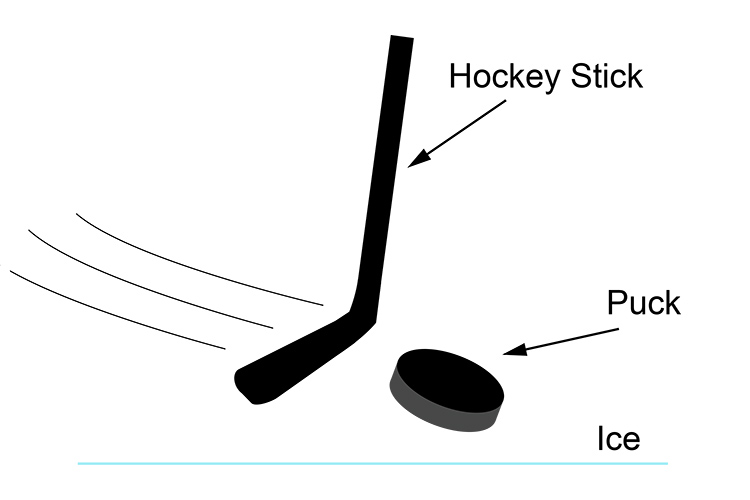An object acted upon by an unbalanced force changes speed and can change direction.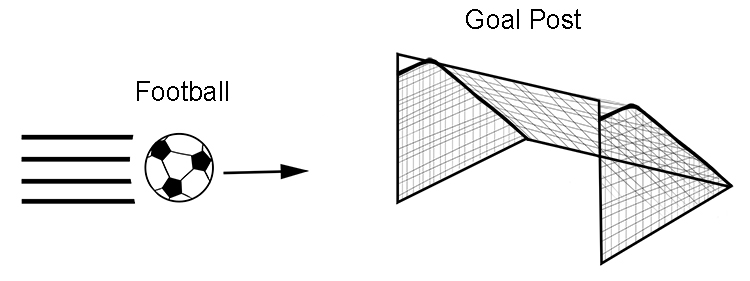An object in motion stays in motion.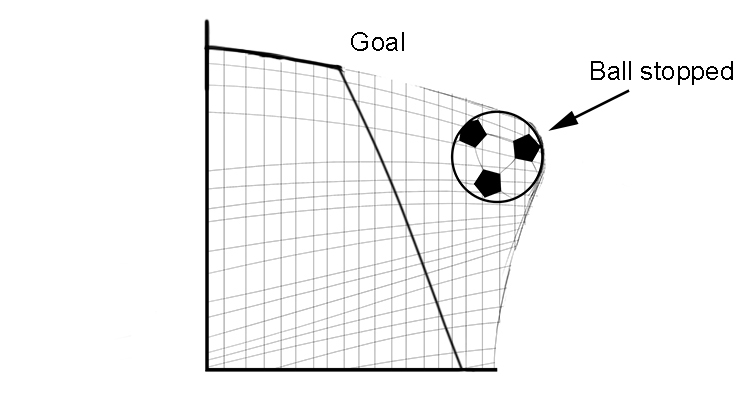An object acted upon by an unbalanced force changes speed and direction.

Example 6

The second part of Newton's first law is sometimes forgotten – you have to remember that it applies to bodies that are moving at a constant velocity, not just those at rest.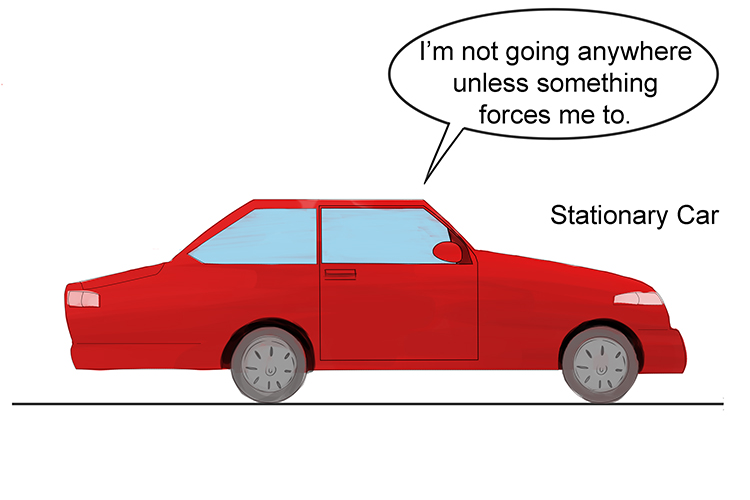This car will never move unless pushed to start moving.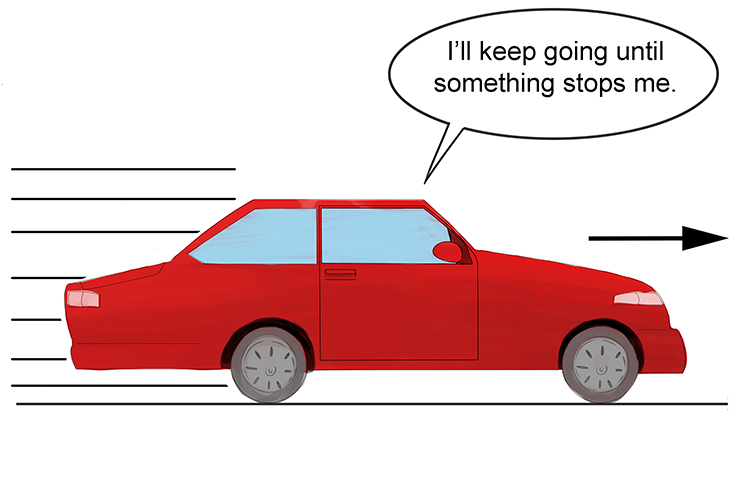This car would never stop unless a force acts upon it. These forces that reduce its speed are friction and drag.

Example 7

Bodies at equilibrium are balanced and therefore there is no acceleration. If all of the forces acting on an object are balanced then the object will continue at the same speed or remain stationary.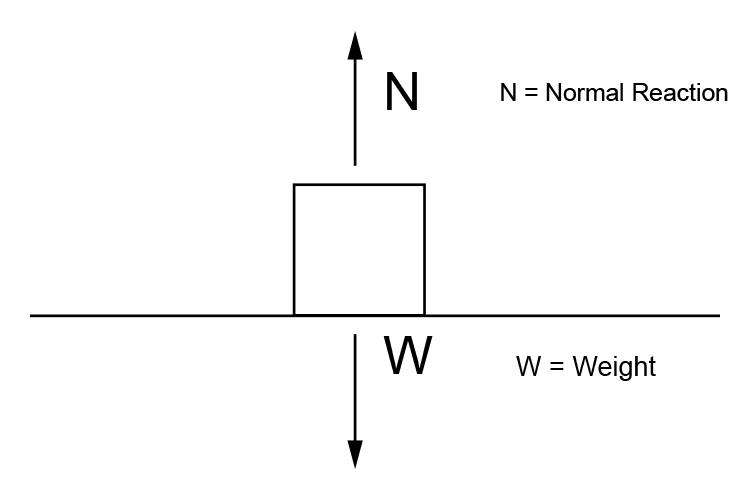N-W=0

N=W

The box is at equilibrium – the sum of all the forces must equal zero.

NOTE: w\e\i\g\h\t=m\a\s\s\xx\g\r\a\v\i\t\y

Example 8

The motion of an aircraft flying through the air can be described by Newton.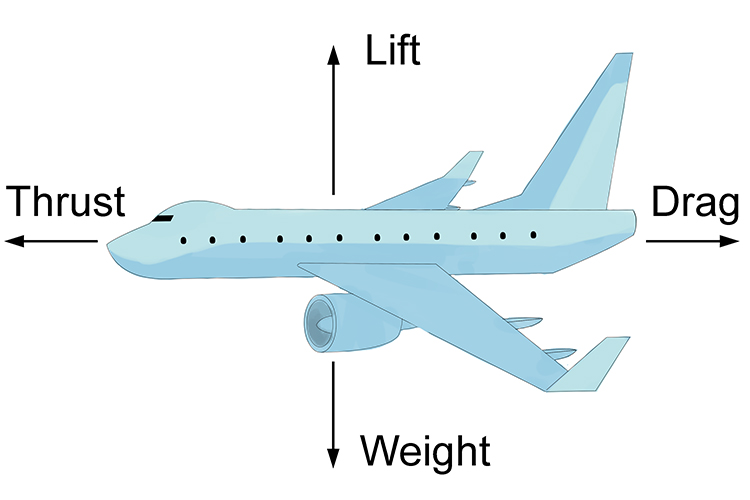If thrust and drag are equal, and lift and weight are also equal, then the aircraft has constant speed and altitude. This means that:

L\i\f\t\=W\e\i\g\h\t

and

D\r\a\g=T\h\r\u\s\t

Example 9

Newton's first law can describe a roller coaster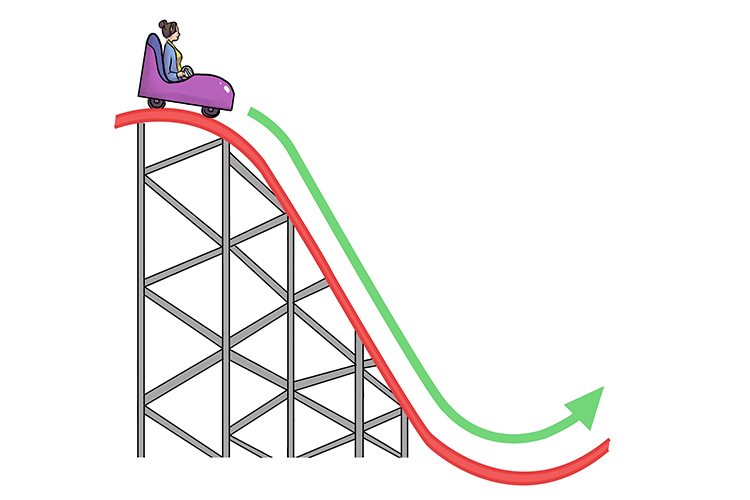A roller coaster has inertia. When it starts a drop it wants to continue moving in the same direction at a constant speed. It doesn't, however, because the tracks act as an outside force that changes the roller coaster's direction.

Example 10

The law of inertia – the tendency for an object to resist changes in motion.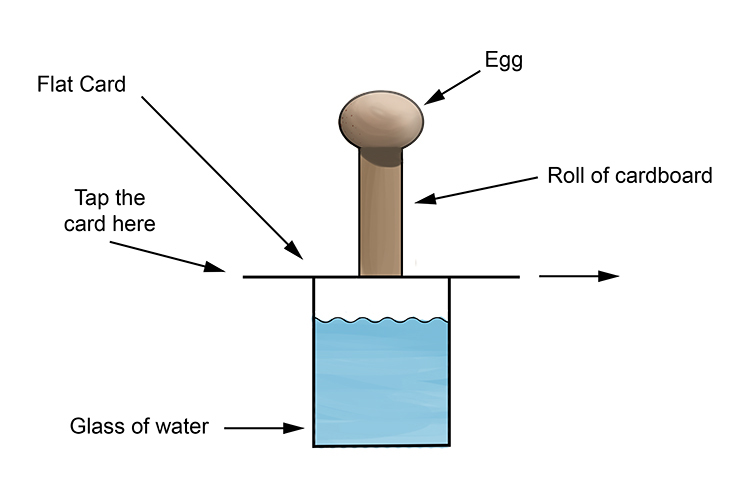Result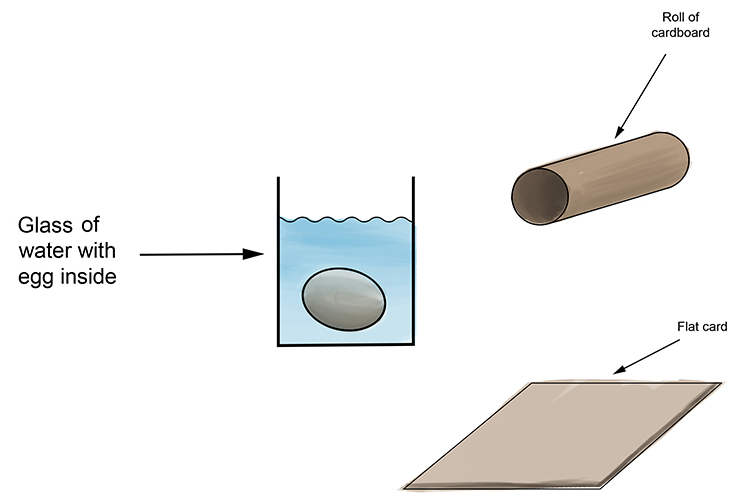The energy of your movement is passed on to the card making it fly out of the way quickly, but the card moves too quickly and there is not enough friction to affect the egg. The egg ends up in the water with nothing holding it up and gravity takes over.

NOTE: This is a similar experiment to pulling a table cloth out from under plates on a table.

Example 11

The movement of a ball in a box can be explained by Newton's first law of inertia.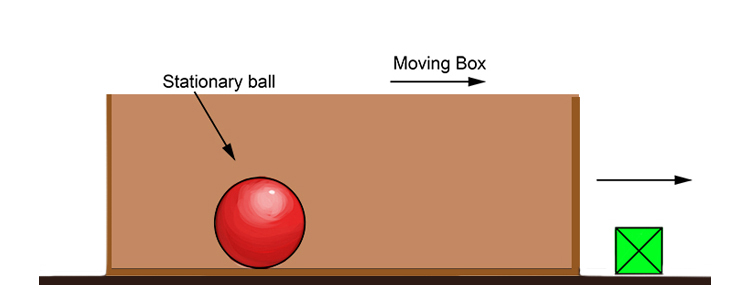Place a ball in a box and slowly push the box.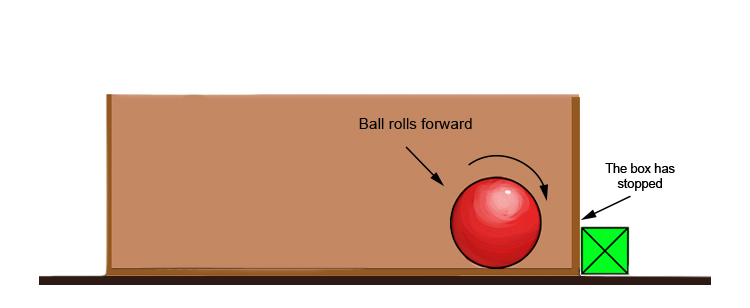Abruptly stop the box. The ball will keep moving. According to Newton's first law, an object in motion tends to stay in motion unless acted upon by an unbalanced outside force, so the ball keeps rolling even though the box has stopped.

Example 12

We put this in for fun and to help you remember the words of Newton's first law of motion.

An object at rest will remain at rest unless acted upon by another force.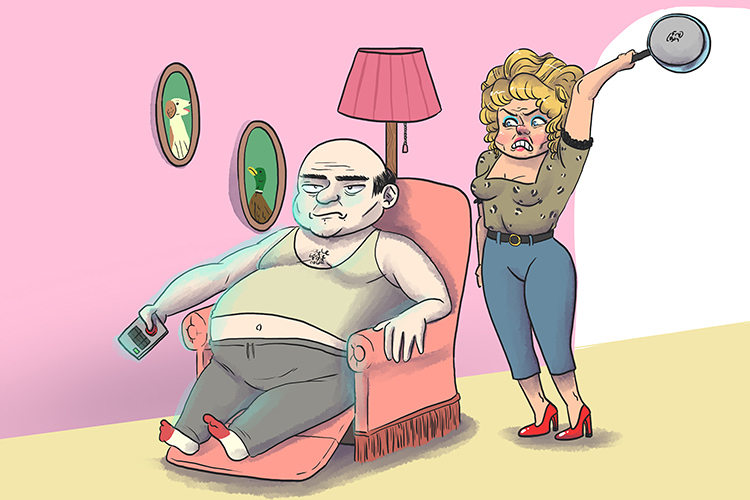Example 13

Galileo observed balls rolling down different curves.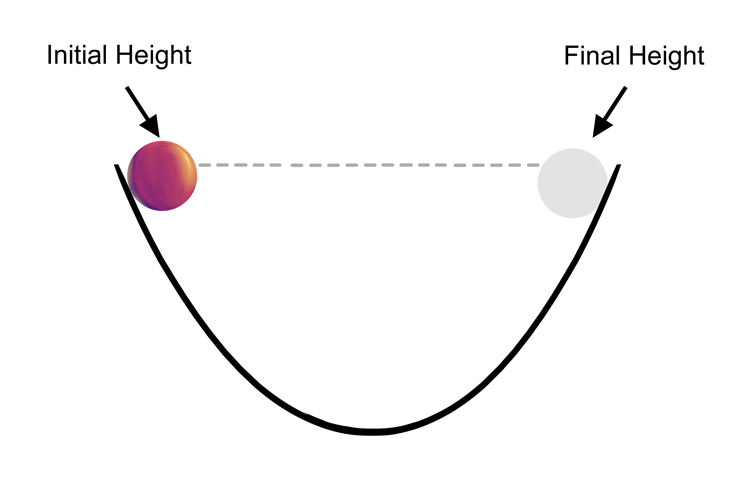The ball will run down one side of the curve then up the other. Galileo noticed that if smooth surfaces were used, the ball got closer to its original height (its height at the starting point). Galileo reasoned that the ball would get to the original height if there were no friction. The ball would get to the original height if there were no outside force (unbalanced force).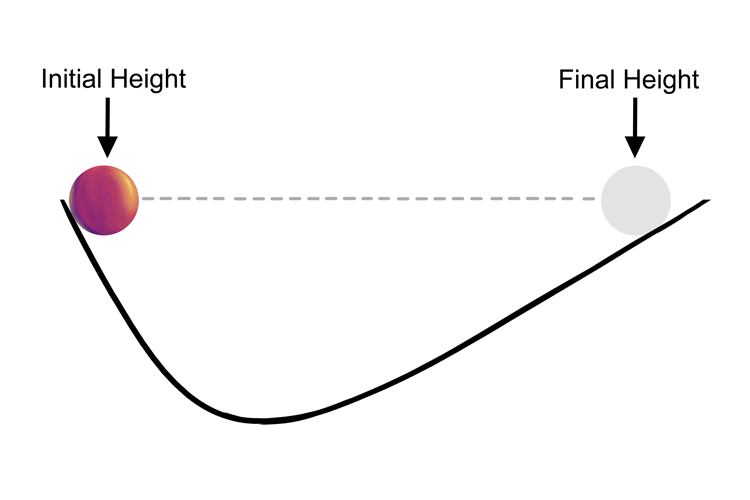Regardless of the angles, the ball will always move towards its original height. If the incline of the second curve is less than that of the first, then the ball will travel a greater distance but still will not reach its original height due to friction – ie, the ball encountering resistance from the surface it is running along. Without friction, the ball would roll up the opposite slope to its original height even if the two ramps had different slopes.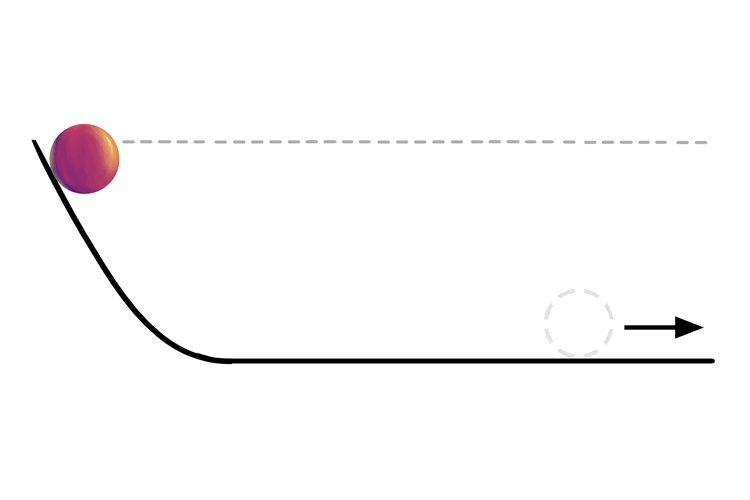Galileo concluded that if the curve ended with no incline then the ball would carry on forever were it not for friction eventually stopping it.

Newton used these observations to conclude that a force is not needed to keep an object in motion. It is in fact an external force that prevents the ball continuing.

What made Newton so radical was that before his first law of motion, scientists had said an object came to a stop because there was no force acting on it.